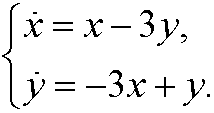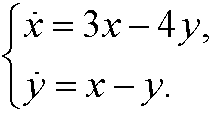# 10. Exercises. ix

9.1) Draw the phase portrait for the system9.2) Draw the phase portrait for the system9.3) Find the general solution and draw the phase portrait for the system9.4) Draw the phase portrait for the system9.5) The equation for a damped harmonic oscillator isRewrite the equation as a system by introducing the variableShow that (0,0) is a critical point. Describe the properties and stability of the critical point in the cases:
a) a=0,

b) a2-4km=0,

c) a2-4km<0,

d) a2-4km>0.

9.6) Describe Verhulst’s population model. In particular describe how this model can be used to illustrate the notions of attractor and chaos. What is Feigenbaum‘s constant?

9.7) Describe how to illustrate Julia sets. What is the famous Mandelbrot set?